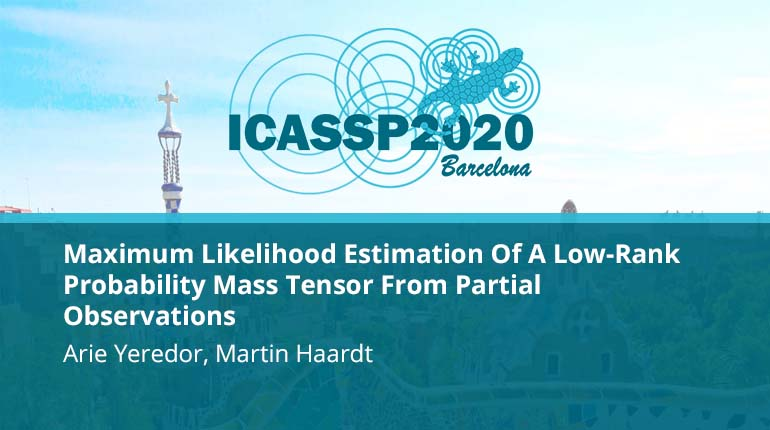This video program is a part of the Premium package:

### Maximum Likelihood Estimation Of A Low-Rank Probability Mass Tensor From Partial Observations

• IEEE MemberUS \$11.00
• Society MemberUS \$0.00
• IEEE Student MemberUS \$11.00
• Non-IEEE MemberUS \$15.00

# Maximum Likelihood Estimation Of A Low-Rank Probability Mass Tensor From Partial Observations

0 views
We consider the estimation of the Probability Mass Function (PMF) of a discrete random vector from partial observations. Since the PMF takes the form of a multi-way tensor, under certain model assumptions the problem becomes closely related to tensor fact
We consider the estimation of the Probability Mass Function (PMF) of a discrete random vector from partial observations. Since the PMF takes the form of a multi-way tensor, under certain model assumptions the problem becomes closely related to tensor fact